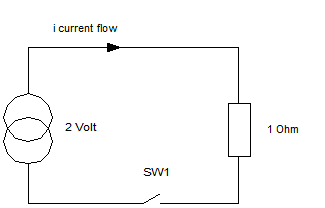# The heating effect of harmonics on supply components

## Current Distortion

A distorted current waveform has higher currents for a shorter period of time so that the same average current flows, but the i squared R component is much higher.

## Simplified Example

Consider this circuit :With a continuous supply voltage of one volt across a constant resistance of one ohm, we have (by ohms law) a continuous current flow of one Amp.

The continuous current flow of 1Amp through a 1 Ohm resister, results in a power dissipation in the resistance of one Watt. Because the current flow is constant, the power dissipated is constant.

## Simplified Example with distorted current

Now, consider this circuit :Case 1. Switch Open:

No current flows, power dissipated = 0 watts.

Case 2. Switch Closed:

With a continuous supply voltage of two volts across a constant resistance of one ohm, we ha a continuous current flow to 2/1 = 2 Amp.

The continous current flow of 2 Amp through a 1 Ohm resister, results in a power dissipation in the resistance of four watts.

Case 3. Switch closed for half the time.

If we now consider the case of the switch closed for 50% of the time, then the average current is 0 amps for half the time, and 2 amps for half the time, average equals 1 Amp.

Under these conditions, the average power dissipated in the resister is 0 watts for half the time, and 4 watts for half the time giving an average power dissipated in the resister of 2 watts.

The "distorted" current waveform has a higher current for a shorter time to yield the same average current, but the power dissipated is much higher due to the current squared power formula. In case three, we have the same average current, but double the average power. - double the power loss.